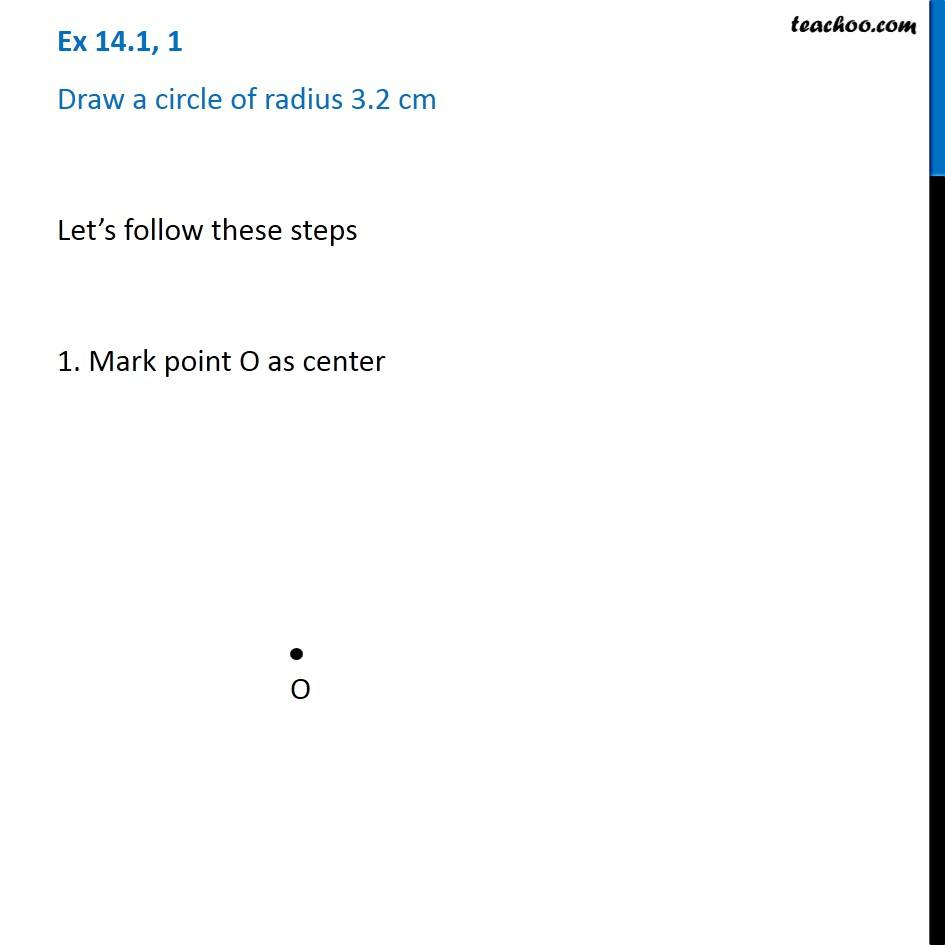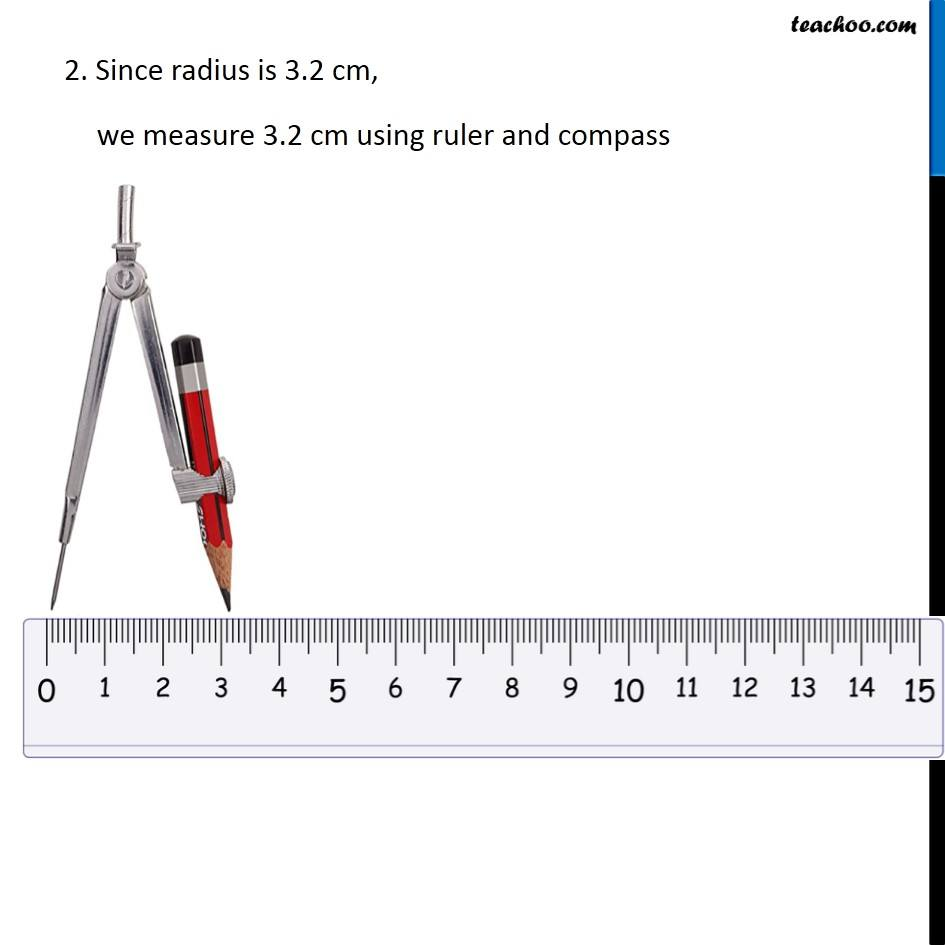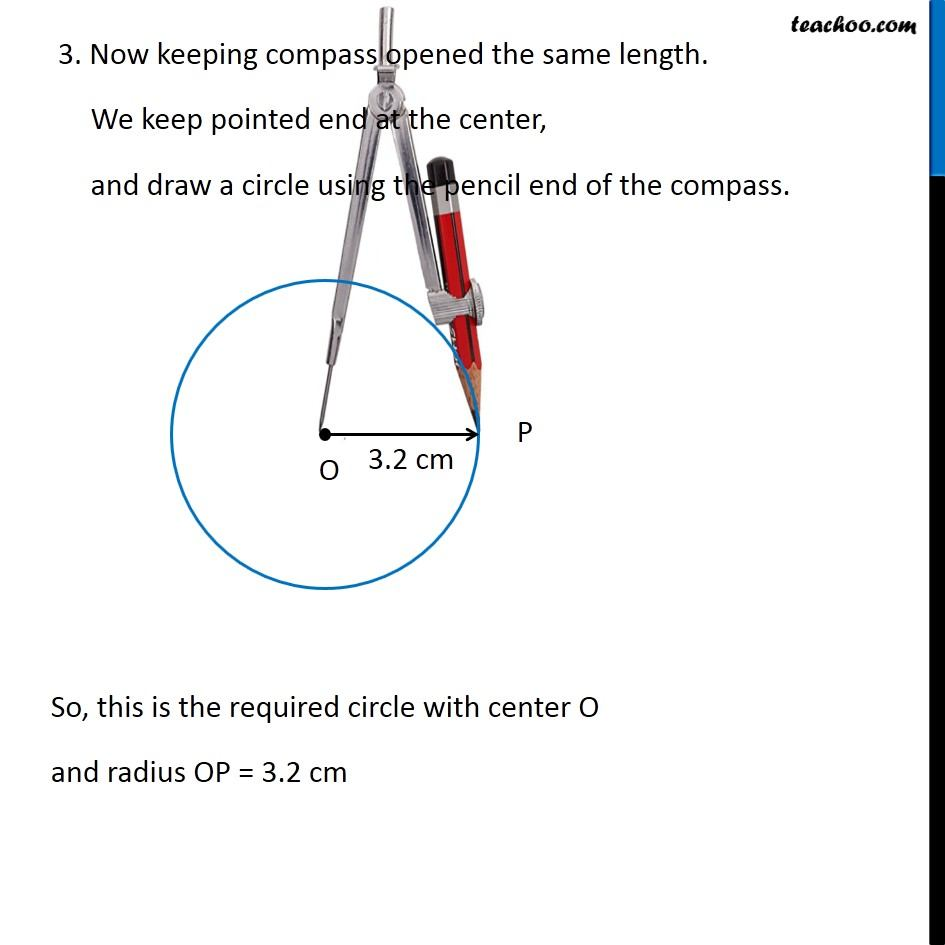Constructing a circle

Practical Geometry
Serial order wiseLearn in your speed, with individual attention - Teachoo Maths 1-on-1 Class

### Transcript

Question 1 Draw a circle of radius 3.2 cm Let’s follow these steps 1. Mark point O as center 2. Since radius is 3.2 cm, we measure 3.2 cm using ruler and compass 3. Now keeping compass opened the same length. We keep pointed end at the center, and draw a circle using the pencil end of the compass. So, this is the required circle with center O and radius OP = 3.2 cm